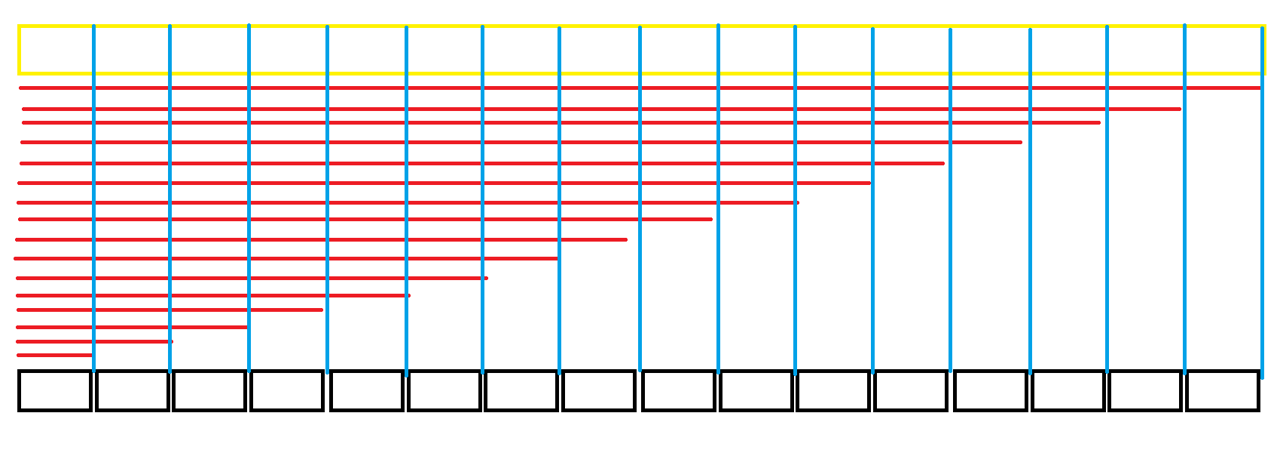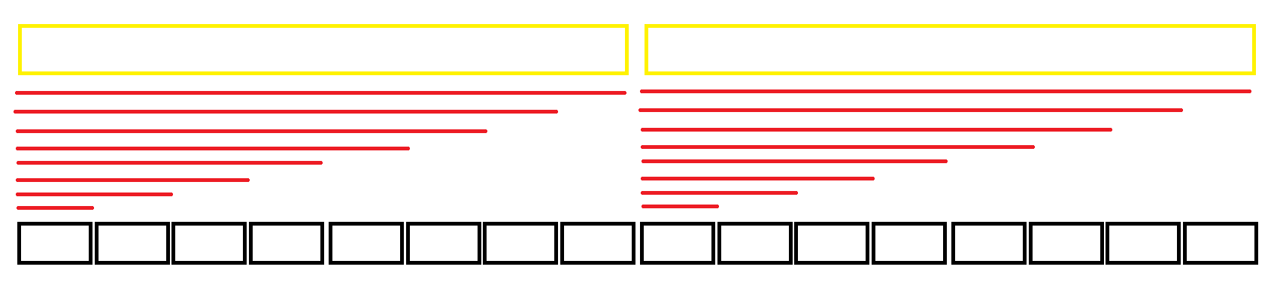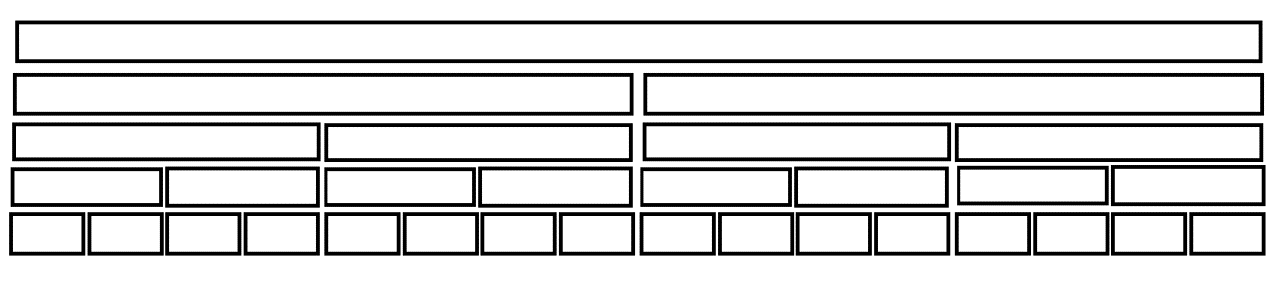线段树一

这里会从我对线段树最开始的理解一点一点的讲下去，

这篇的查询问题均为区间查询, 修改问题均为单点修改sum[r] - sum[l - 1] = \sum_{i = l}^r a[i]struct node
{
int l, r, val;
//我们可以这样定义对于当前结点所包含的区间信息为, 区间[l, r]的和为val
}tr[length * 4]; // *4是为了符合可以完整的建立起来这棵树,而且不会出现数组越界之类的情况
//这个length可以不为2^k的倍数

void build(int k, int l, int r) // 当前建立的结点是区间l到r的,是编号为k的结点
{
tr[k].l = l;
tr[k].r = r;
if(l = r)
{
tr[k].val = a[l]; // 第k个结点对应的叶子结点储存的值
return ; // 结束递归
}
int mid = (l + r) / 2;
build(k * 2, l, mid);
// 先建立并填写完整左子树的信息,至于为什么是k * 2之类的可以先复习一下二叉树的知识点
build(k * 2 + 1, mid + 1, r);
//同上
tr[k].val = tr[k * 2].val + tr[k * 2 + 1].val;
//递归的维护信息
}//只有先递归到叶子结点维护好叶子结点信息之后在一层一层的往上依次维护就可以简单的维护完成
void change(int k, int l, int r, int val) // 这里的操作是单点加,其他操作都大同小异
{
// 其实只需要a[k].l == a[k].r就行,单点修改的l和r本身就相等
if(l <= a[k].l && a[k].r <= r)
{
tr[k].val += val;
return ; //结束递归
}
int mid = (a[k].l + a[k].r) / 2; // 这里是a[k].l和a[k].r不是l和r
if(l <= mid) change(k * 2, l, r, val);
else change(k * 2 + 1, l, r, val);
//单点操作非左即右
tr[k].val = tr[k * 2].val + tr[k * 2 + 1].val; // 这句话不能忘写
}
// 这个函数就不写为什么可以这样写了
int query(int k, int l, int r)
{
if(l <= a[k].l && a[k].r <= r)
{
return a[k].val;
}
int mid = (a[k].l + a[k].r) / 2;
int ans = 0;
if(l <= mid) ans += query(k * 2, l, r);
if(r >  mid) ans += query(k * 2 + 1, l, r);
return ans;
}